# Diffraction gratings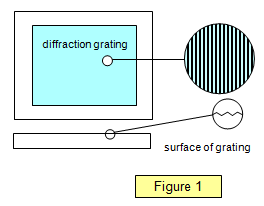If the number of slits in an obstacle is large the sharpness of the pattern is improved, the maxima getting narrower. Obstacles with a large number of slits (more than, say, 20 to the millimetre) are called diffraction gratings. These were first developed by Fraunhofer in the late eighteenth century and they consisted of fine silver wire wound on two parallel screws giving about 30 obstacles to the millimetre.

Since then many improvements have been made, in 1882 Rowland used a diamond to rule fine lines on glass, the ridges acting as the slits and the rulings as the obstacles (See Figure 1). Using this method it is possible to obtain diffraction gratings with as many as 3000 lines per millimetre although 'coarse' gratings with about 500 lines per millimetre are better for general use.

In many schools two types are in common use, one with 300 lines per mm and the other with 80 lines per mm.
Reflection gratings are also used, where the diffracted image is viewed after reflection from a ruled surface. A very good example of a reflection diffraction grating is a CD. A DVD with finer rulings gives a much broader diffraction pattern.

## The wave theory and the diffraction grating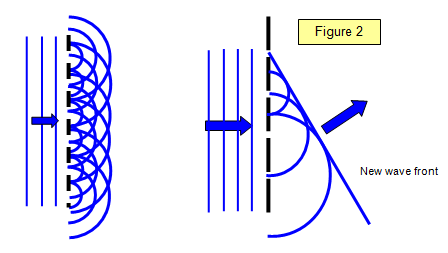Figure 2 shows the Huygens construction for a grating. You can see how the circular diffracted waves from each slit add together in certain directions to give a diffracted wave which has a plane wave front just like the waves hitting the grating from the left. This plane wave is formed by drawing the line that meets all the small circular waves and is called an envelope of all these small secondary waves.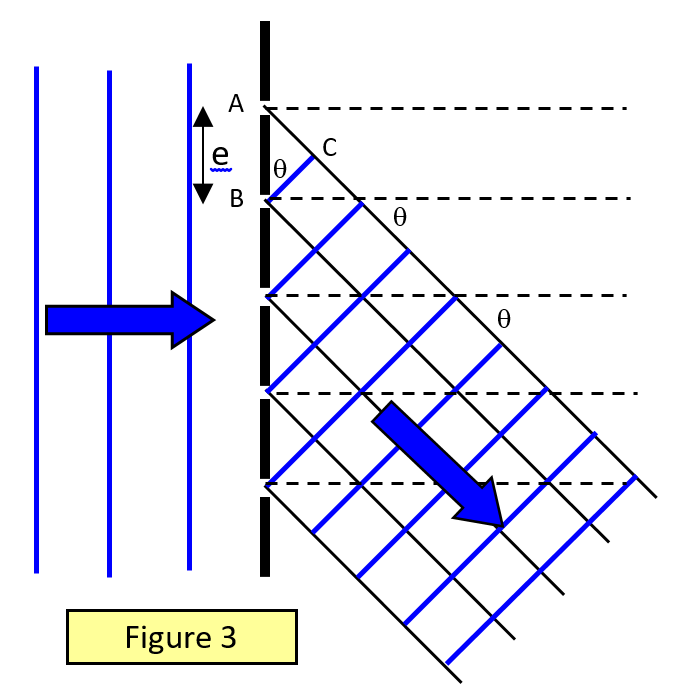## The diffraction grating formula

Consider a parallel beam of light incident normally on a diffraction grating with a grating spacing e (the grating spacing is the inverse of the number of lines per unit length). Consider light that is diffracted at an angle q to the normal and coming from corresponding points on adjacent slits (Figure 3).
For a maximum the path difference = AC = mλ
But AC = e sinθ. Therefore for a maximum:

Diffraction grating maximum
mλ = e sinθ
where m = 0, 1, 2,3...

The number m is known as the order of the spectrum, that is, a first-order spectrum is formed for m = 1, and so on.

If light of a single wavelength, such as that from a laser, is used, then a series of sharp lines occur, one line to each order of the spectrum. With a white light source a series of spectra is formed with the light of the shortest wavelength having the smallest angle of diffraction.

In deriving the formula above, we assumed that the incident beam is at right angles to the face of the grating. Allowance must be made if this is not the case. The simplest way is to measure the position of the first order spectrum on either side of the centre, record the angle between these positions and then halve it, as shown in Figure 4.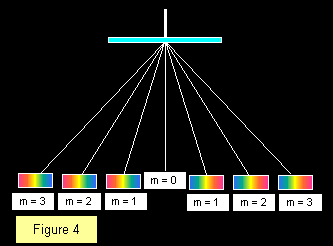The number of orders of spectra visible with a given grating depends on the grating spacing, more spectra being visible with coarser gratings. The ruled face of the grating should always point away from the incident light to prevent errors due to changes of direction because of refraction in the glass. The diagram shows a central white fringe with three spectra on either side giving a total of seven images.

(See example problem )

Example problems
1. Calculate the wavelength of the monochromatic light where the second order image is diffracted through an angle of 25o using a diffraction grating with 300 lines per millimetre.
Grating spacing (e) = 10-3/300 m = 3.3x10-6 m
Wavelength (l) = esin25/2 = [3.3x10-6 x 0.42]/2 = 6.97x10-7 m = 697 nm

2. Calculate the maximum number of orders visible with a diffraction grating of 500 lines per millimetre, using light of wavelength 600 nm.
Maximum angle of diffraction = 90o e = 10-3/500 = 2x10-6 m
Therefore m = esinq/l = 2x10-6/600x10-9 = 3.33
Therefore maximum number of orders = 3, and a total of seven images of the source can be seen (three on each side of a central image).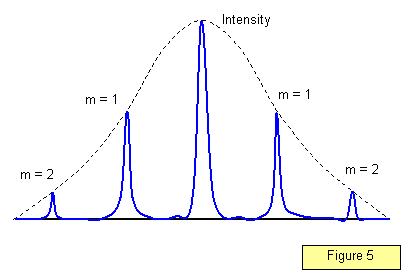The intensity distribution in the diffraction pattern for a large number of slits is shown in Figure 5. Notice that the maxima become much sharper; the greater the number of slits per metre, the better defined are the maxima.

Student investigation
The diffraction of cadmium or mercury light is used to determine the separation of two lines on an integrated circuit. The following results were obtained for the second order diffracted images for different wavelengths. Use them to plot an appropriate linear graph and thence determine the mean spacing of the wires on the circuit.

 Wavelength/nm Angle of diffraction (o) 468 28.0 480 28.7 509 31.0 546 33.0 577 35.5 644 40.0